Z scores and Confidence Intervals

STANDARDIZED VALUE: The concept of least squares is a property of the mean. It essentially says to take the actual values measured and subtract the mean; then square this value. Do this for every member of a population and add all the derived squares. The result is called the sum of least squares. Mean derivation gives an optimum starting place to get the smallest (sigma). This means the standard deviation computed for a set of scores is less than any other measure of variation. Since all normal distributions are the same except for their order of magnitude, a value can be standardized by subtracting the mean and dividing by the standard deviation.

Z = (X - µ)/ or (X -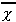)/s for a sample.

Where Z is a standardized score. X is value of the case for that variable. is the standard deviation of the population. s is the standard deviation of the sample.(pronounced X bar) is the mean of sample. (pronounced muu) is the mean of the population.

CONFIDENCE INTERVALS & SAMPLE SIZE

CONFIDENCE INTERVAL OF SAMPLE MEANS: a univariate inferential statistic for estimating the range of the population mean at a certain confidence level using interval data.

C.I. =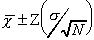where: = the standard deviation for the population

N = the total number of cases in the sample= the sample mean average

Z = standardized score of chosen probability level

assumption: N 100

In most cases an estimate of the standard error (or bias) of the sample will be needed. This is done by using the standard deviation of the sample in place of the population standard deviation and subtracting 1 from N.

C.I. =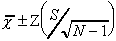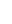Example: What is the confidence interval for Introductory Social Statistics class averages at a probability level of .05? The sample mean is 72.76, the standard deviation is 15.48 and the sample total is 150.

C.I. =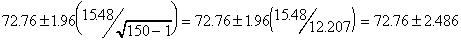Interpretation: There is a 95% chance that an Introductory Social Statistics class average falls between 70.27 and 75.25.

If the total sample size is increased, the estimated standard error of the means (the sample bias) becomes smaller and the interval becomes narrower.

Example: change the above example's total to 1,500

C.I. =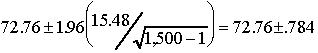CONFIDENCE INTERVALS OF PROPORTIONS: univariate inferential statistic for estimating the range of proportions for the population from a sample proportion using nominal data.

C.I. =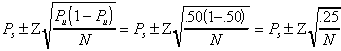Where: Ps = Sample proportion

Pu = Population proportion

N = Sample total

Z = Standardized Score of chosen probability level

Assumptions:

N > 100

Random sample

Once again, all values are known except one -- Pu. Because the population proportion is not known, it is estimated by substituting the maximum possible value. This will achieve the most conservative estimate of the interval or sample bias.

Example:

 Do you approve of Bill Clinton's actions as President of the United States? RELATIVE PROPORTION YES 416 .416 NO 389 .389 UNDECIDED 195 .195 TOTAL 1,000

Q: If the probability level is .01, what is the margin of error if the proportion of people in the U. S. that approve of Bill Clinton's actions as President of the United States is estimated?

A:   C.I. =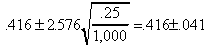With a margin of error of + four percentage points, 42% of the people in the U. S. approve of Bill Clinton's actions as President of the United States.

*NOTE: The 4% percentage point bias can describe all proportions of answers in the sample. With a margin of error of + four percentage points, 39% of the people in the U. S. do not approve of Bill Clinton's actions as President of the United States.

ESTIMATE SAMPLE SIZE

Using this same formula, researchers are able to estimate what size the sample must be in order to have a certain margin of error. Most polls allow for a 3 percentage point margin of error with a probability level of 5%. Both the probability level and size of the sample influence the outcome. Larger samples have narrower interval. Smaller probability levels (possibility of being wrong) increases the width of the interval.

Q: What size of random sample would a researcher need if the margin of error desired was 3 percentage points while maintaining a confidence level of 95%?

A: C.I. = .03 Z = 1.96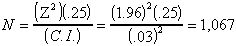The sample size should be 1,067.